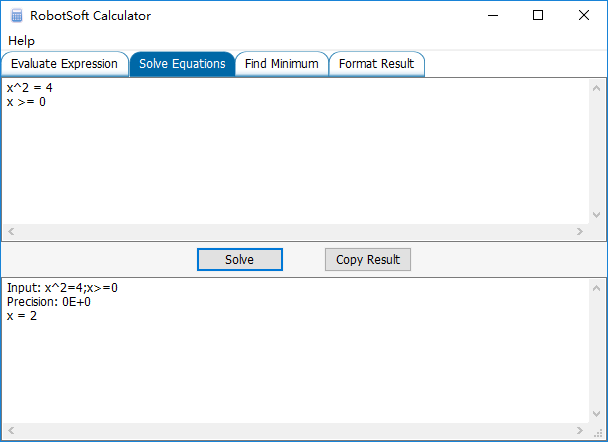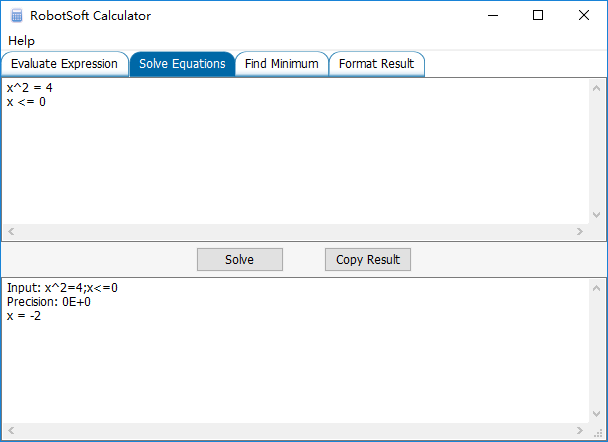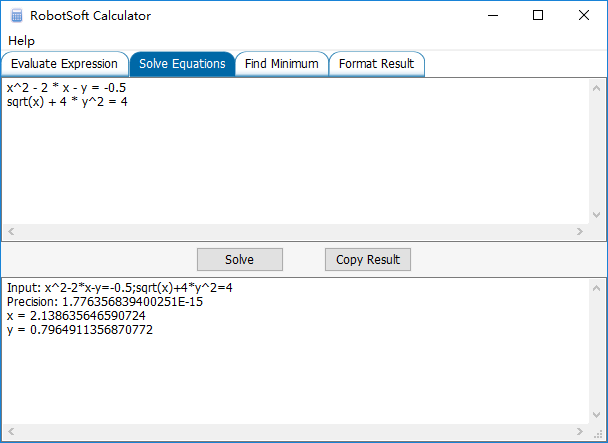## RobotSoft Calculator now supports Greater Equal and Less Equal command

Uncategorized Comments Off on RobotSoft Calculator now supports Greater Equal and Less Equal command
Nov 192016

The Solve Equations feature of RobotSoft Calculator now supports Greater Equal and Less Equal command. Different conditions may get different results.

Assume x >= 0:Assume x <= 0:## Solve complex nonlinear equations with RobotSoft Calculator

Uncategorized Comments Off on Solve complex nonlinear equations with RobotSoft Calculator
Nov 172016

RobotSoft Calculator is an advanced calculator which can solve nonlinear equations easily.